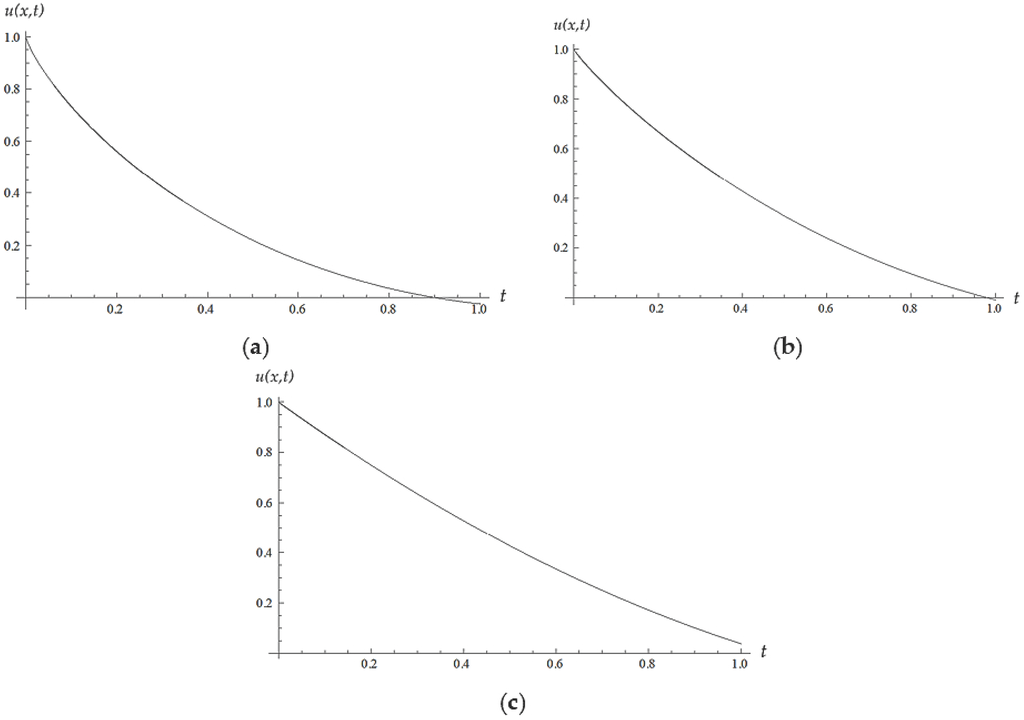Previous Article in Journal
Network Entropies of the Chinese Financial Market
Previous Article in Special Issue
Analytical Solutions of the Electrical RLC Circuit via Liouville–Caputo Operators with Local and Non-Local Kernels
Open AccessArticle

# Solution of Higher Order Nonlinear Time-Fractional Reaction Diffusion Equation

byNeeraj Kumar Tripathi 1,†,Subir Das 1,†,Seng Huat Ong 2,†,Hossein Jafari 3,4,*,† andMaysaa Al Qurashi 5,†1
Department of Mathematical Sciences, Indian Institute of Technology (BHU), Varanasi 221 005, India
2
Institute of Mathematical Sciences, University of Malaya, Kuala Lumpur 50603, Malaysia
3
Department of Mathematical Sciences, University of South Africa, UNISA, Pretoria 0003, South Africa
4
Department of Mathematics, University of Mazandaran, Babolsar 47416, Iran
5
Department of Mathematics, King Saud University, Riyadh 11495, Saudi Arabia
*
Author to whom correspondence should be addressed.
Entropy 2016, 18(9), 329; https://doi.org/10.3390/e18090329
Received: 8 July 2016 / Revised: 30 August 2016 / Accepted: 31 August 2016 / Published: 8 September 2016
(This article belongs to the Special Issue Wavelets, Fractals and Information Theory II)
The approximate analytical solution of fractional order, nonlinear, reaction differential equations, namely the nonlinear diffusion equations, with a given initial condition, is obtained by using the homotopy analysis method. As a demonstration of a good mathematical model, the present article gives graphical presentations of the effect of the reaction terms on the solution profile for various anomalous exponents of particular cases, to predict damping of the field variable. Numerical computations of the convergence control parameter, used to evaluate the convergence of approximate series solution through minimizing error, are also presented graphically for these cases. View Full-Text
Show FiguresFigure 1

MDPI and ACS Style

Tripathi, N.K.; Das, S.; Ong, S.H.; Jafari, H.; Al Qurashi, M. Solution of Higher Order Nonlinear Time-Fractional Reaction Diffusion Equation. Entropy 2016, 18, 329.